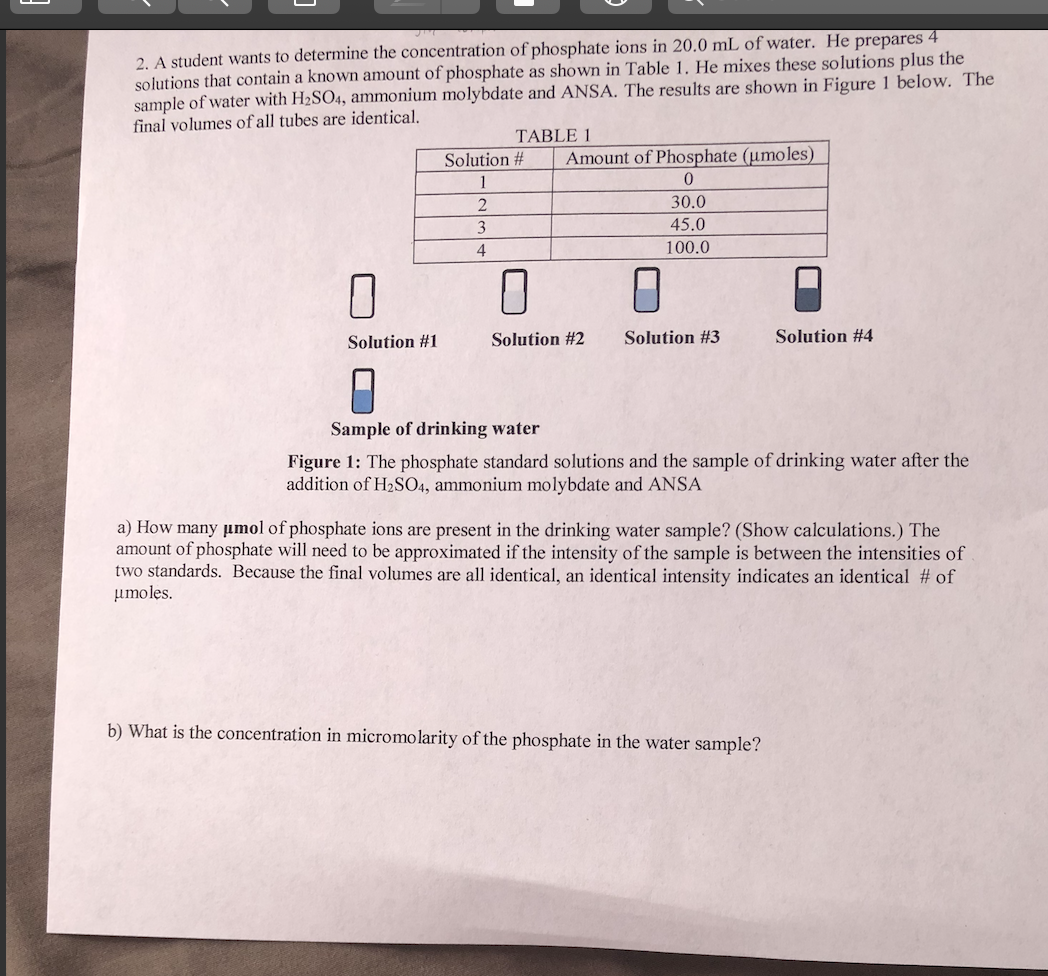# 2. A student wants to determine the concentration of phosphate ions in 20.0 mL of water. He prepares 4 solutions that contain a known amount of phosphate as shown in Table 1. He mixes these solutions plus the sample of water with HzSO4, ammonium molybdate and ANSA. The results are shown in Figure 1 below. The final volumes of all tubes are identical. TABLE1 | Amount of Phosphate (umoles) 0 30.0 45.0 100.0 Solution # 4 Solution #1 Solution #2 Solution #3 Solution #4 Sample of drinking water Figure 1: The phosphate standard solutions and the sample of drinking water after the addition of H2SO4, ammonium molybdate and ANSA a) How many umol of phosphate ions are present in the drinking water sample? (Show calculations.) The amount of phosphate will need to be approximated if the intensity of the sample is between the intensities of two standards. Because the final volumes are all identical, an identical intensity indicates an identical # of umoles. b) What is the concentration in micromolarity of the phosphate in the water sample?

Question

Im having difficulty with part A and B of this homework problem, thanks in advancehelp_outlineImage Transcriptionclose2. A student wants to determine the concentration of phosphate ions in 20.0 mL of water. He prepares 4 solutions that contain a known amount of phosphate as shown in Table 1. He mixes these solutions plus the sample of water with HzSO4, ammonium molybdate and ANSA. The results are shown in Figure 1 below. The final volumes of all tubes are identical. TABLE1 | Amount of Phosphate (umoles) 0 30.0 45.0 100.0 Solution # 4 Solution #1 Solution #2 Solution #3 Solution #4 Sample of drinking water Figure 1: The phosphate standard solutions and the sample of drinking water after the addition of H2SO4, ammonium molybdate and ANSA a) How many umol of phosphate ions are present in the drinking water sample? (Show calculations.) The amount of phosphate will need to be approximated if the intensity of the sample is between the intensities of two standards. Because the final volumes are all identical, an identical intensity indicates an identical # of umoles. b) What is the concentration in micromolarity of the phosphate in the water sample? fullscreen

1 Rating

### Want to see this answer and more?

Experts are waiting 24/7 to provide step-by-step solutions in as fast as 30 minutes!*

*Response times vary by subject and question complexity. Median response time is 34 minutes and may be longer for new subjects.
Tagged in
ScienceChemistry

### Solutions